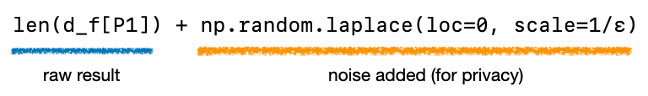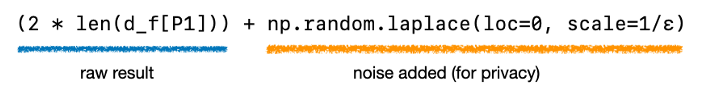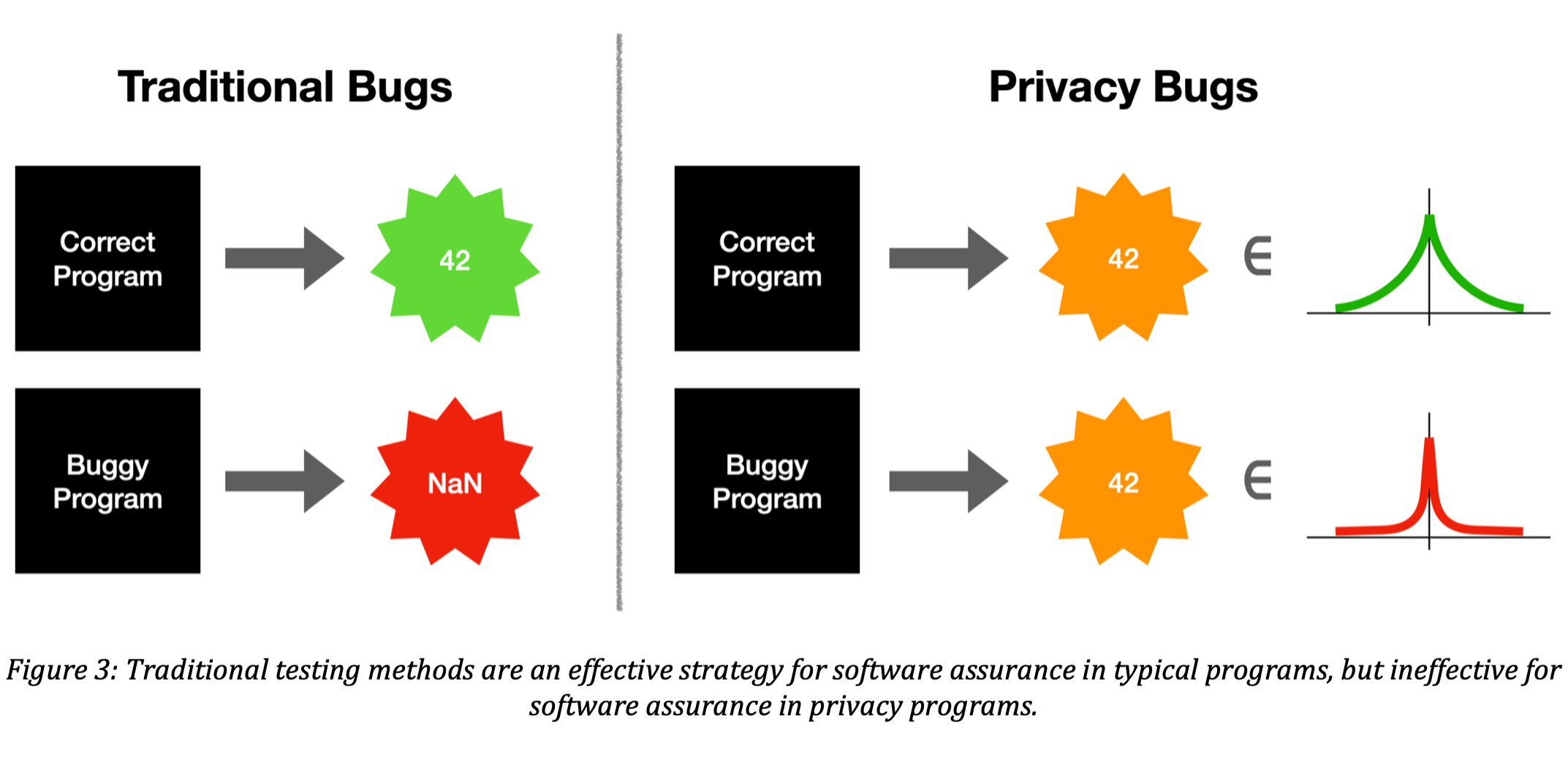# Differential Privacy Bugs and Why They’re Hard to FindCredit: metamorworks/shutterstock.com

In previous posts we have explored what differential privacy is, how it works, and how to answer questions about data in ways that protect privacy. All of the algorithms we’ve discussed have been demonstrated via mathematical proof to be effective for protecting privacy. However, when translating these algorithms from paper to code, it’s possible to introduce bugs in the resulting software which can result in failure to protect privacy. In this post we’ll explore what these bugs typically look like, why it is so hard to detect them, and approaches to software assurance that can ensure your implementation is free from bugs.

## What Does a Privacy Bug Look Like?

What does a “privacy bug” look like when implementing differentially private algorithms? Remember that the central idea of differential privacy is to add noise to data analysis results in order to protect privacy. When a certain quantity of noise is added, a certain quantity of privacy is achieved. Privacy bugs happen when too little noise is added, resulting in too little achieved privacy, or when too much noise is added, resulting in too much degradation of the result’s accuracy.

Recall our previous post on counting queries, where we implemented a differentially private count of elements in a dataset as:The scale parameter is where we choose how much noise to add to the raw result len(d_f[P1]). Because the sensitivity of the raw result is 1, and we choose the scale of 1/ε when generating Laplace noise, we know the result will satisfy ε-differential privacy.

Now, what if wanted to compute 2 * len(d_f[P1]) (i.e., 2 times the previous result) in a differentially private way? Let’s try it out:It turns out this algorithm doesn’t implement ε-differential privacy. Why? Because the raw result is now 2-sensitive instead of 1-sensitive. As written, it satisfies ε/2-differential privacy, and if we wanted to achieve ε-differential privacy, we would have to change the scale parameter to 2/ε.In general, to achieve ε-differential privacy for an s-sensitive function, you must add Laplace noise with scale s/ε to the result.

Two common classes of privacy bugs for programs which use differential privacy are:

1. Adding the wrong amount of noise (like the example above), and
2. Incorrectly calculating the sensitivity of a function.

Both of these bugs are hard to avoid 100% of the time. Later in this post we’ll discuss some tools that can help catch these types of errors.

Note that these are not the only sources of bugs in differentially private algorithms – mistakes are easy to make in other areas that are challenging to catch. For example, failures of privacy can arise from buggy proofs , details of floating-point arithmetic , problematic random-number generators , and even the program’s execution time .

## Why is Catching Privacy Bugs So Hard?

The typical method for catching software bugs is testing. A test case has two parts: an example input we want to test the program on, and an example output that we expect the function to compute on that input. For example, if we implemented an efficient integer square-root operation in code, we might test it on (4,2), (9,3) and (16,4) as example input/output pairs. However, testing is only as useful as the test cases you try it on. What if we forgot to test (1,1)? What should the function do on inputs 0, or -1? (Should it crash? Return an error code?) We can’t be sure that any program is “100% correct” until we test it on all possible inputs, and this in general isn’t possible if there are too many possible inputs (e.g., trillions, or infinitely many).

Despite its limitations, testing is the leading approach for software assurance in practice. This is because most correctness criteria can be captured surprisingly effectively by enumerating expected input/output pairs. However, as we will see, traditional testing approaches are not effective for catching privacy bugs. This is because differentially private programs provide randomized outputs, and privacy bugs are not detectable by observing output values; instead, they are only detectable by observing output distributions, as shown in the following figure.On the left of the figure is the traditional testing scenario for software bugs. We can run correct programs and observe good output, like 42, and we can run buggy programs and observe bad output, like NaN. On the right is the traditional testing scenario applied to privacy bugs. A correct program and a buggy program could both return 42, making it hard to discern which program is buggy just by looking at the output. The difference is that the good program’s output of 42 is drawn from a “good” distribution, whereas the bad program’s output of 42 is drawn from a “bad” distribution. Each output is drawn from the Laplace distribution, but with a different scale parameter. A bad scale parameter is indicative of the privacy bug, but this cannot be seen after observing just one draw from the distribution.

Let’s dive a little deeper into why the first type of privacy bug—adding incorrect noise—is hard to identify via testing. (The second type of privacy bug—incorrect sensitivity calculation—is also hard to identify via testing, but the details are more technical, so we will just focus on adding incorrect noise in this post, and touch on this second class of bugs in a future post.) In the buggy program example we forgot to change the scale parameter, and this bug changes the distribution of results changes in a very subtle yet problematic way. Here are some sample output values when running the buggy example program 10 times (where scale = 1/ε), versus running the correct program 10 times (where scale = 2/ε). Both runs assume the raw result is 2000 and that ε is set to 1. The results are displayed in a random order, and the difference is that the buggy program only satisfies ε/2 differential privacy, whereas the correct program satisfies ε differential privacy. Can you tell which one is which?

Results A

1999.1804565506698

2002.7147480061415

2000.6802541404093

1999.4774430452733

1998.867213030664

2001.0902402006432

2002.085675105062

2001.1242404052532

1999.6440121715966

2000.7331674628506

Results B

1999.9695117126153

1999.7900561829326

1996.7327340370953

2003.5572723890255

2000.7025617293127

2001.369585666838

1999.8483501006692

1996.3832196985252

2001.5842721434444

2001.6591643136965

The answer is that Results A are from the buggy program, and Results B are from the correct program, despite Results A providing only half the guarantee of privacy. In general, it’s nearly impossible to tell how much privacy an algorithm gives by looking at one or a small handful of output values. It actually would be possible to tell which output came from the buggy program if we looked at hundreds or thousands of samples, but this requires knowing exactly what the sensitivity of the algorithm is, and therefore what scaling to expect in the output distribution.

## How Do We Catch Privacy Bugs?

So, if traditional testing techniques are not very helpful for discovering privacy bugs, how are we to achieve software assurance, and gain confidence that we have implemented differential privacy correctly? There are two classes of approaches for this, each of which are custom-tailored for differential privacy: (1) sophisticated testing frameworks, and (2) sophisticated program analysis. Both of these approaches are topics of ongoing research, and we are just starting to see practical tools emerge from the research groups that investigate this problem.

In terms of sophisticated testing, there are a number of approaches [1,2], for example, Ding et al  employ a statistical hypothesis testing approach which is able to detect privacy bugs for small programs in a matter of seconds. In terms of program analysis, there are also a number of approaches [3,4,5] which vary in their support for automation and programming language features. We expect to see practical tools appear in the next few years for providing assurance that programs which implement differential privacy are free of privacy bugs, based on either sophisticated testing or program analysis frameworks.

## Coming Up Next

In the next post, we’ll dig deeper into techniques for testing differentially private programs. These techniques run the program many times on many different inputs and analyze the results to catch the elusive bugs we just described. These techniques are developing quickly and are an important part of ensuring correctness as differential privacy is transitioned from theory to practice.

This post is part of a series on differential privacy. Learn more and browse all the posts published to date on the differential privacy blog series page in NIST’s Privacy Engineering Collaboration Space.

Source link